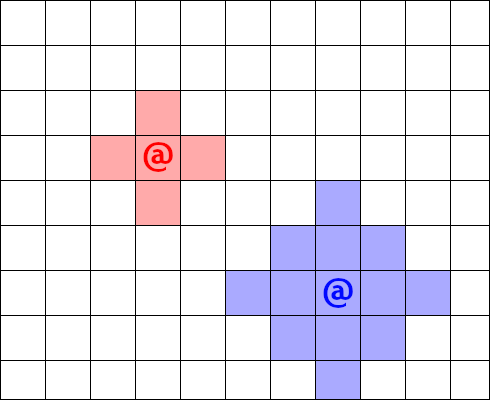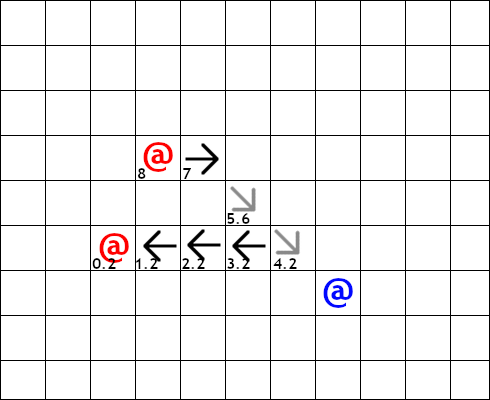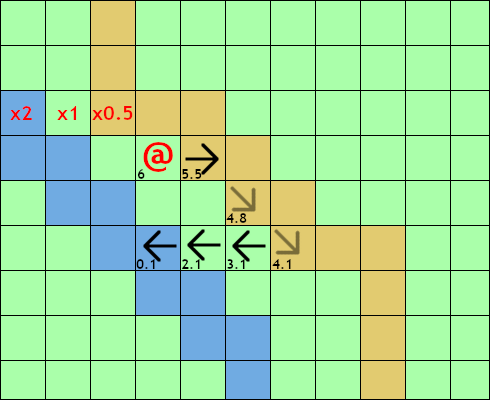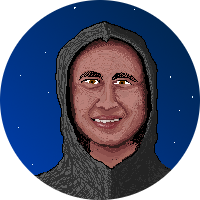/ #mechanics

# Movement: Costs

In real life and real-time video games, movement takes time. In turn-based games, time is abstracted so we also need to abstract the fact that movement costs time.

This can be approached in several ways, described in some more detail below.

Simple

A unit can move once per turn, but only up to a maximum distance based on its speed.

For example in the diagram below the red character has a speed of 1, so can only move into the red tinged spaces for the turn; the blue character has a speed of two so it can reach a few more spaces.Fixed Cost

A unit can move multiple times during its turn as long as it has movement points. It receives movement points equal to its speed every turn and every move costs a fixed amount of points. Usually costs are based on the Euclidean distance travelled, which works out to a cost of 1 for an orthogonal moves and 1.4 for a diagonal move.

The example below shows the movement points of the red character at each position. There might be an interaction with the blue character while next to it, but this does not cost movement points in this example.Variable Cost

Works the same as fixed cost, but each map tile has a different terrain type that multiplies the movement cost.

The example below has grass which doesn’t modify movement costs, dirt roads which halve movement cost and water which doubles movement costs.Some examples of these different modes in use include Heroes of Might and Magic, which uses the simple method during battles and variable cost method on the world map. Chaos Battle of Wizards used the fixed cost method since the map was just an abstract grid without any terrain types.

## ECS Design

This ECS design example is for the most complex case, which is variable movement cost. You might notice that this essentially the grid-based movement ECS design, with a few small changes to account for the movement costs.

### Components

#### Mover

• move_points: Float
• move_speed: Float

• x: Integer
• y: Integer

#### Tile

• x: Integer
• y: Integer
• passable: Boolean
• cost_multiplier: Float

#### MoveCommand

• delta_x: Integer (-1, 0, 1)
• delta_y: Integer (-1, 0, 1)

#### SelectedUnit

Marker component for marking the selected unit.

### Entities

One entity per map tile in the grid with a Tile component.

One Position entity per unit in the game with Position component.

### Systems

#### InputSystem

The InputSystem gathers input from the user and turns it into MoveCommand components on the unit being moved.

``````key = get_keypresses()
selected_entity = world.get_entity_with_component(SelectedUnit)

while not valid_input(key):
# Only orthogonal movement implemented in this example
if key == LEFT:
elif key == RIGHT:
elif key == UP:
elif key == DOWN:

key = get_keypresses()
``````

#### MovementSystem

The MovementSystem applies movement commands to the entity if terrain and movement points allow.

``````for entity in world.get_entities_with_components(Mover, Position, MoveCommand):
target_x = entity.position.x + entity.move_command.delta_x
target_y = entity.position.y + entity.move_command.delta_y

target_tile = world.get_tile_at(target_x, target_y)
# Practically speaking to do the above line efficiently
# you actually need some map data structure in addition
# to the ECS entities for the tiles...

delta_x = target_tile.x - entity.position.x
delta_y = target_tile.y - entity.position.y
distance = sqrt(delta_x * delta_x + delta_y * delta_y)
move_cost = distance * target_tile.cost_multiplier
if not target_tile.passable:
message("You walk into the wall.")
elif not entity.mover.move_points >= move_cost:
message("You do not have enough movement points left to reach there...")
else:
entity.position.x = target_x
entity.position.y = target_y
entity.mover.move_points -= move_cost

world.remove_component(entity, MoveCommand))
``````#### Matt Van Der Westhuizen

Back-end service developer at Ubisoft Blue Byte by day - wannabe game designer & developer by night.